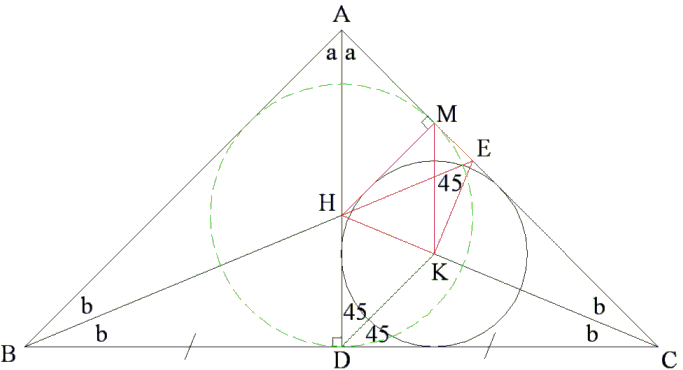## Problem 4 from the IMO 2009

Here is Problem 4 from the IMO 2009:

 Let ABC be a triangle with AB = AC. The angle bisectors of ∠CAB and ∠ABC meet the sides BC and CA at D and E, respectively. Let K be the incentre of triangle ADC. Suppose that ∠BEK = 45°. Find all possible values of ∠CAB.

Solution### Solution by Vo Duc Dien (Saturday July 18, 2009) Dedicated to my teacher Nguyen Hoi

Extend CK to meet BE at H. H is the center of the incircle of triangle ABC. HM = HD.Since CH bisects ∠ACB, the two right triangles HDC and HMC are equal. Therefore ∠HMK = ∠HDK = 45°.

There are two possibilities: either M and E coincide or they are different. In the former case, BM is both the median and the altitude from B, implying BC = AB. Since also, AB = AC, all three sides in ΔABC are equal and the triangle is equilateral. Thus ∠CAB = 60°.

If M and E are distinct, we recall that ∠HEK = ∠BEK = 45°. Thus the quadrilateral HMEK is cyclic and, since ∠HME = 90°, HE serves as its diameter. It follows that ∠HKE is also right so that ∠KHE = 45°.

Further, ∠BHC = 180° - ∠KHE = 135°, 2b = 45°.

In triangle ABC we have ∠CAB = 180° - 4b = 90° which is the second possible value for ∠CAB.